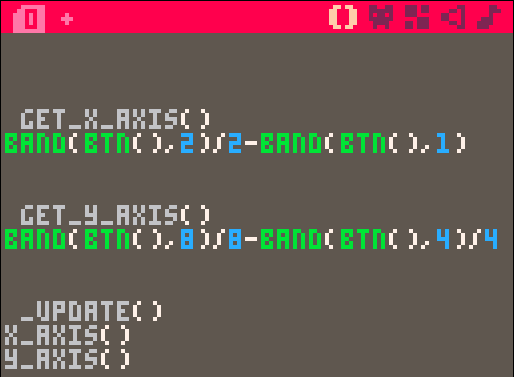# Responding to Buttons using Bitwise Addition in PICO-8It is possible to respond to each button press in PICO-8 by using conditional statements like this:

```if btn(0) then vx=-1 end
if btn(1) then vx=1 end
if btn(2) then vy=-1 end
if btn(3) then vy=1 end```

But a more elegant solution is to use the bitwise addition function band().  Calling btn() with no arguments results in a bitfield of outputs.  In other words, pressing button 1 (right) gives 000010.

Continuing with the example of pressing the right button, the result is 000010 and so looking at the code in get_x_axis():

`band(btn(),2)/2-band(btn(),1)`

band(000010,2)/2 returns 1
band(000010,1) returns 0

so 1-0 returns 1, i.e. move to the right (positive).

By checking the bits and subtracting the right from left (or down from up) we end up with a value of -1 or 1 for the movement.

I’ve wrapped the bitwise calculations here into two functions for neatness (get_x_axis and get_y_axis), but this is slightly profligate with tokens and by directly referencing the band() function you can reduce your token count by two from the standard conditional method shown above.

```vx=0
vy=0

function get_x_axis()
return band(btn(),2)/2-band(btn(),1)
end

function get_y_axis()
return band(btn(),8)/8-band(btn(),4)/4
end

function _update()
vx=get_x_axis()
vy=get_y_axis()
end

function _draw()
cls()
print(vx,5,5)
print(vy,5,11)
end```

Feel free to use, and if you spot any further optimizations, let me know!